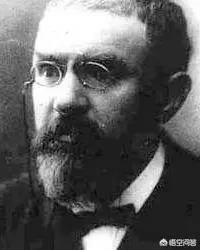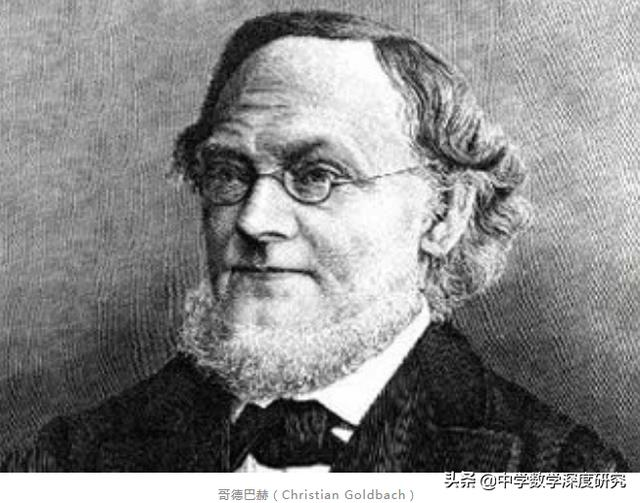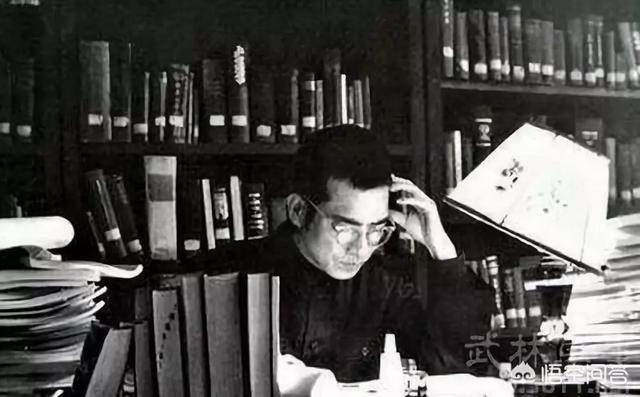# 哥德巴赫猜想如果被证明了，对数学、对科学、对生活会有什么意义？（引入原文）孪生素数公式

（1）.两孪生素数，：例如3和5 ，5和7，11和13，…，

(2).三孪生素数，例如41.43.和47 ，461.463.和467，613.和617.619，…，

（3）四孪生素数，例如11.13.和17.19 ，101.103.和107.109，821.823.和827.829，…，，

（4）头孪生素数，例如a1087.1089a1091a，a1867a1871 1873p.1877 1879a ，a7207 a7211 7213a，…，

（5）尾孪生素数，例如a1607 1609a1613a，a2657 2659a2663a，a8861 8863a 8867a a8969 8971a ，…

（6）头尾孪生素数，例如a1087 a1091 1093a 1097a

，a1423a1427.1429a1433a，a1297 a1301 1303a 1307a，…，，

（部分）

10…10=5q5.12=7q5.14=7q7.16=11q5.18=11q7.20=13q7.

22=11q11.24=11w13.26=13q13.28=17q11.3

1000.1000=569q431.1002=569q433.1004=571q433.1006=857q149.

1008=857q151.1010=829q181.1012=821q191.1014=191q823.

1016=193q823.1018=419q599.1020=1019q1.1022.=1021q1.

1024=1021q3.1026=1021q5.1028=1021q7.1030=853q277.

1032=1031q1.1034=1033q1.1036=1033q3.1038=1033q5. pppp

1040=1033q7.1042=521q321.1044=033q11.1146=433q613.

1048=857q191.1050=1033q17.1052=1033q19.1054=857q197.

1056=857q199.1058=601q457.1060=1049q11.1062=1033q29.

1064=1033q31.1066=467q599.1068=467q601.1070=457q613.

1072=431q641.1074=1033q41.1076

9148=137q9011.9150=137q9013.9152=139q9013.9154=113m9041.

9156=113m9043.9158=619q8539.9160=149q9011.9162=149q9013.

9164=151q9013.9166=197q8969.9168=197q8971.917

## “数学皇冠上的明珠”——哥德巴赫猜想

1742年6月7日,德国数学家哥德巴赫（C．Goldbach，1690-1764）在给大数学家欧拉的信中提出了两个关于正整数与素数之间关系的推猜:

1) 每一个不小于６的偶数都是两个奇素数之和．

2) 每一个不小于９的奇数都是三个奇素数之和．这就是有名的哥德巴赫猜想,第一个通常被叫做”关于偶数的哥德巴赫猜想”，而另一个被称为”关于奇数的哥德巴赫猜想”。因为任何一个不小于9的奇数都可以写成一个不小于6的偶数与3的和,于是,如果关于偶数的哥德巴赫猜想成立,那么关于奇数的哥德巴赫猜想也是成立的.因此,现在提哥德巴赫猜想,通常是指关于奇数的猜想.## 研究状况

1742年6月30日欧拉给哥德巴赫回信。这个命题看来是正确的，但是他也给不出严格的证明。同时欧拉又提出了另一加强版本（想想为什么是加强的）：任何一个大于2的偶数都是两个素数之和。但是这个命题他也没能给予证明。今日常见的猜想陈述为欧拉的版本。而哥德巴赫的原问题被称为弱哥德巴赫猜想，已于2013年被巴黎高等师范学院研究员哈洛德·贺欧夫各特证明。

1920年，挪威的布朗证明了“9 + 9”。

1924年，德国的拉特马赫证明了“7 + 7”。

1932年，英国的埃斯特曼证明了“6 + 6”。

1937年，意大利的蕾西先后证明了“5 + 7”，“4 + 9”，“3 + 15”和“2 + 366”。

1938年，苏联的布赫夕太勃证明了“5 + 5”。

1940年，苏联的布赫夕太勃证明了“4 + 4”。

1956年，中国的王元证明了“3 + 4”。稍后证明了“3 + 3”和“2 + 3”。

1948年，匈牙利的瑞尼证明了“1 + c”，其中c是一很大的自然数。

1962年，我国的潘承洞和苏联的巴尔巴恩证明了“1 + 5”， 中国的王元证明了“1 + 4”。

1965年，苏联的布赫 夕太勃和小维诺格拉多夫，及意大利的朋比利证明了“1 + 3”。

1966年，我国的陈景润证明了“1 + 2”成立，即“任一充分大的偶数都可以表示成两个素数之和，或是一个素数和一个半素数之和”，距离“1 + 1”仅“一步之遥”。这是目前这一研究方向的最好结果。关于陈景润证明“1 + 2”的故事，可参考距“哥猜”最近的数学家——陈景润。2000年3月18日，美英两家出版社联合宣布：谁能在两年内解开“哥德巴赫猜想”这一古老的数学之谜，可以得到100万美元的奖金。这再次使“哥德巴赫猜想”成为社会关注的热点，还引得无数的“民间数学家”为此孜孜不倦地努力。两年早过去了，尽管国内外有不少人如何热衷于破解此难题，国内有许多人甚至言辞凿凿地说自己破解了哥德巴赫猜想，以至于中科院数学与系统科学所的院士们每天都能接到全国各地的电话或来信，甚至还有人千里迢迢带着自己的草稿守在中科院数学与系统科学所门口，一些人拿着猜想的“最终证明结果”轮流拜访多位数学家，也不时传出“农民成功证明哥德巴赫猜想”、“拖拉机手摘得‘皇冠上的明珠’”等“爆炸性新闻”,但并没有人能领取到这笔奖金.

## 搞事情!那些不能说的秘密都在这里   关注公众号：科普Room### 觉得文章有用就打赏一下文章作者

#### 支付宝扫一扫打赏#### 微信扫一扫打赏